# How to Calculate and Solve for Reservoir Radius and Well Spacing | The Calculator Encyclopedia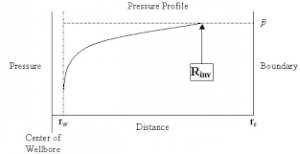The image above represents the reservoir radius.

To compute for the reservoir radius, one essential parameter is needed and this parameter is Well Spacing (A).

The formula for calculating the reservoir radius:

re = √[43560A  / π]

Where;

A = Well Spacing

Let’s solve an example;
Given that the well spacing of an reservoir radius is 42. Find the reservoir radius?

This implies that;

A = Well Spacing = 42

re = √[43560A  / π]
re = √[43560 x 42 / π]
re = √[1829520 / π]
re = √582354.3
re = 763.12

Therefore, the reservoir radius is 763.12 ft.

Calculating the well spacing (A) when the Reservoir Radius is Given.

A = π x re2 / 43560

Where;

A = Well Spacing

Let’s solve an example;
Find the well spacing when the reservoir radius is 60.

This implies that;

re = Reservoir Radius = 60

A = π x re2 / 43560
A = π x 602 / 43560
A = π x 3600 / 43560
A = 11309.73 / 43560
A = 0.259

Therefore, the well spacing is 0.259.

Nickzom Calculator – The Calculator Encyclopedia is capable of calculating the reservoir radius.

To get the answer and workings of the reservoir radius using the Nickzom Calculator – The Calculator Encyclopedia. First, you need to obtain the app.

You can get this app via any of these means:

To get access to the professional version via web, you need to register and subscribe for NGN 1,500 per annum to have utter access to all functionalities.
You can also try the demo version via https://www.nickzom.org/calculator

Apple (Paid) – https://itunes.apple.com/us/app/nickzom-calculator/id1331162702?mt=8
Once, you have obtained the calculator encyclopedia app, proceed to the Calculator Map, then click on Petroleum under EngineeringNow, Click on Reservoir Fluid Flow under Petroleum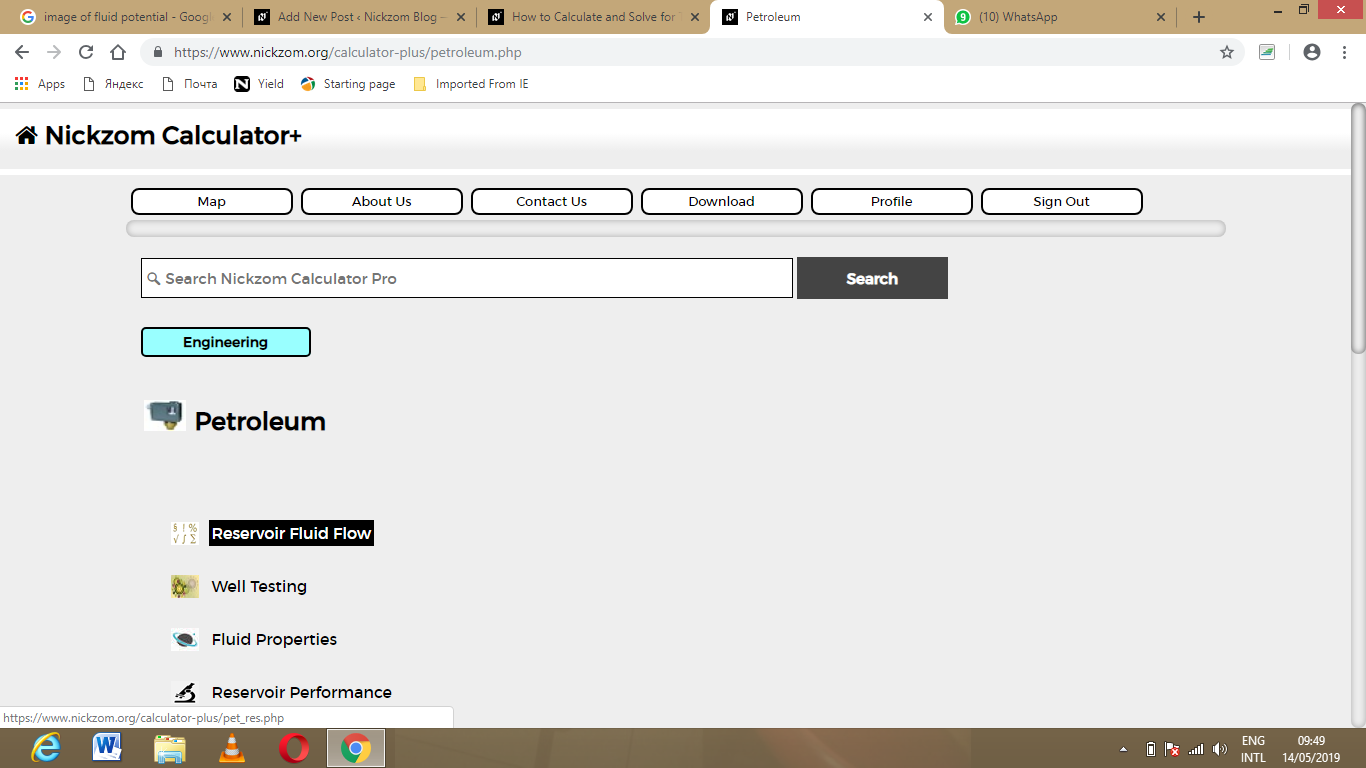Now, Click on Reservoir Radius under Reservoir Fluid Flow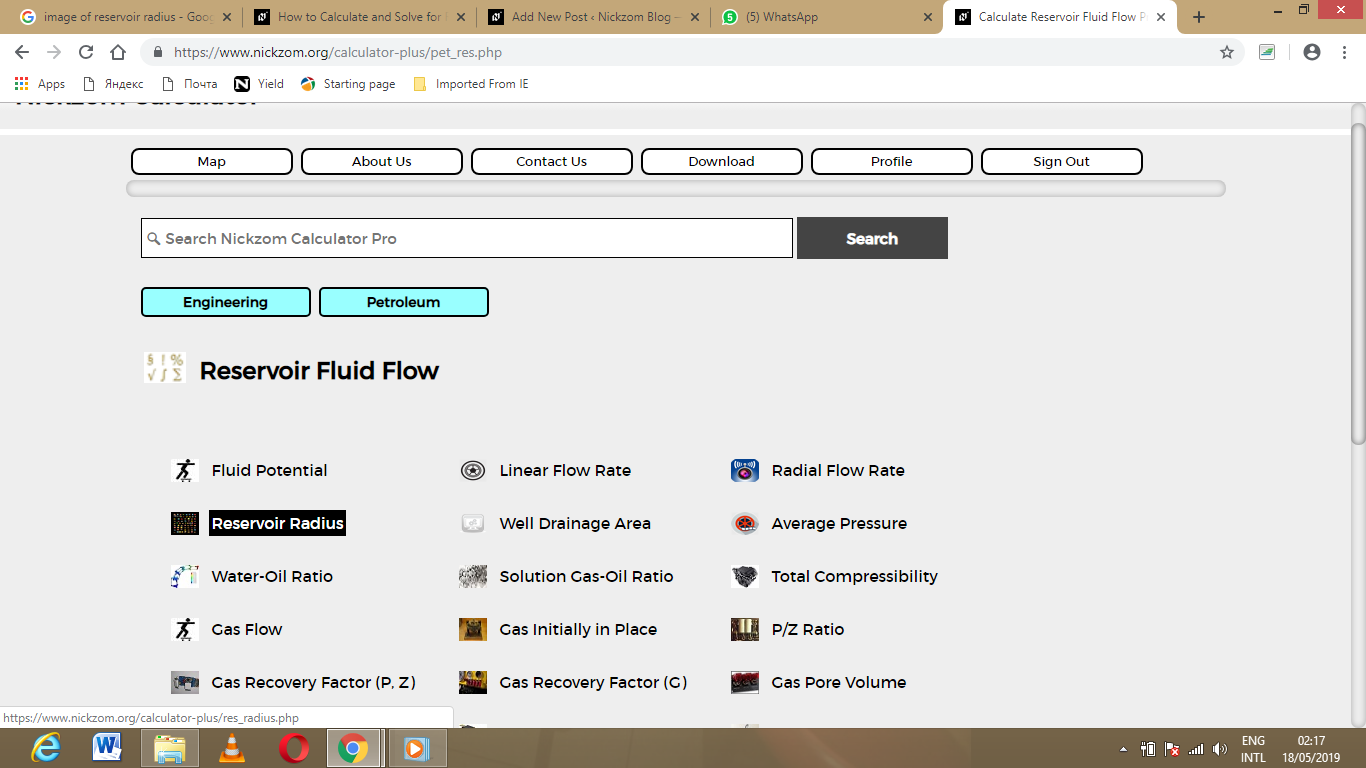The screenshot below displays the page or activity to enter your value, to get the answer for the reservoir radius according to the respective parameter which are the well spacing (A).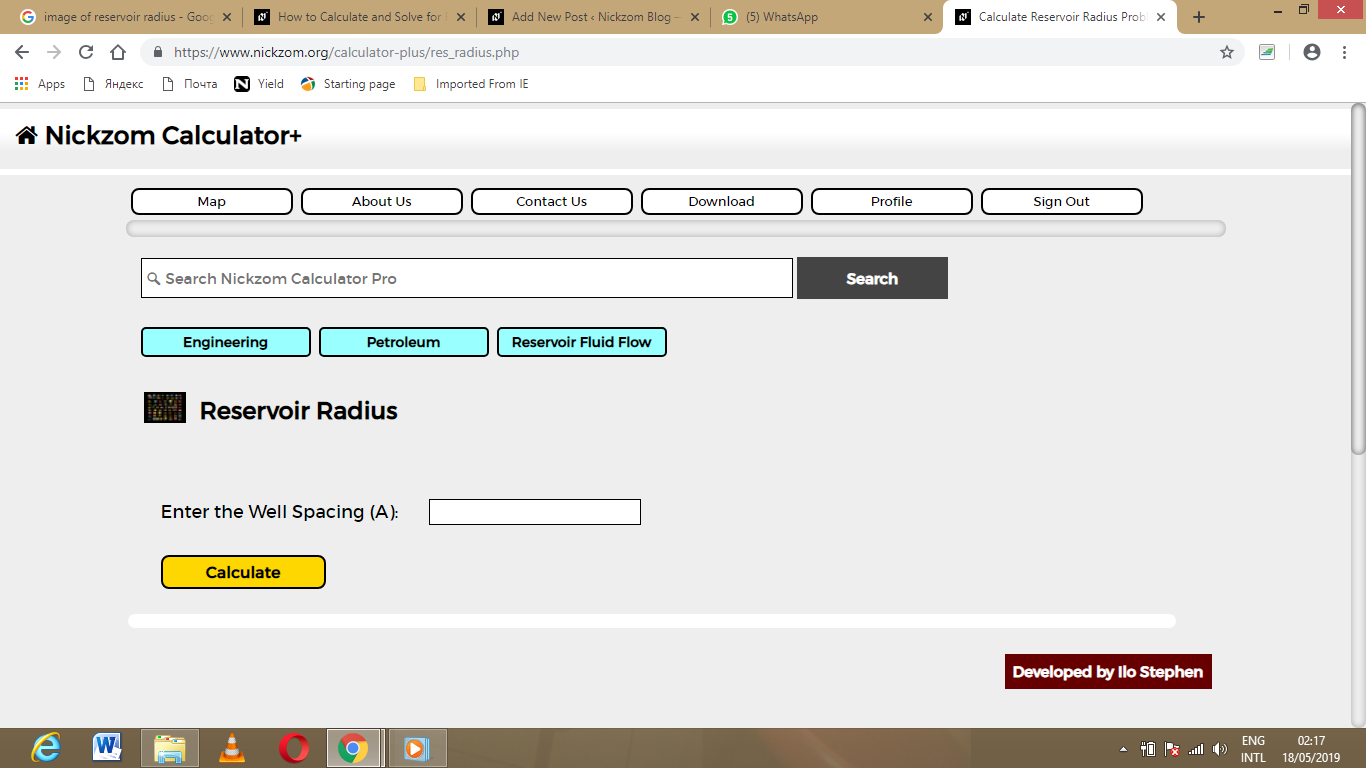Now, enter the value appropriately and accordingly for the parameter as required by the well spacing (A) is 42.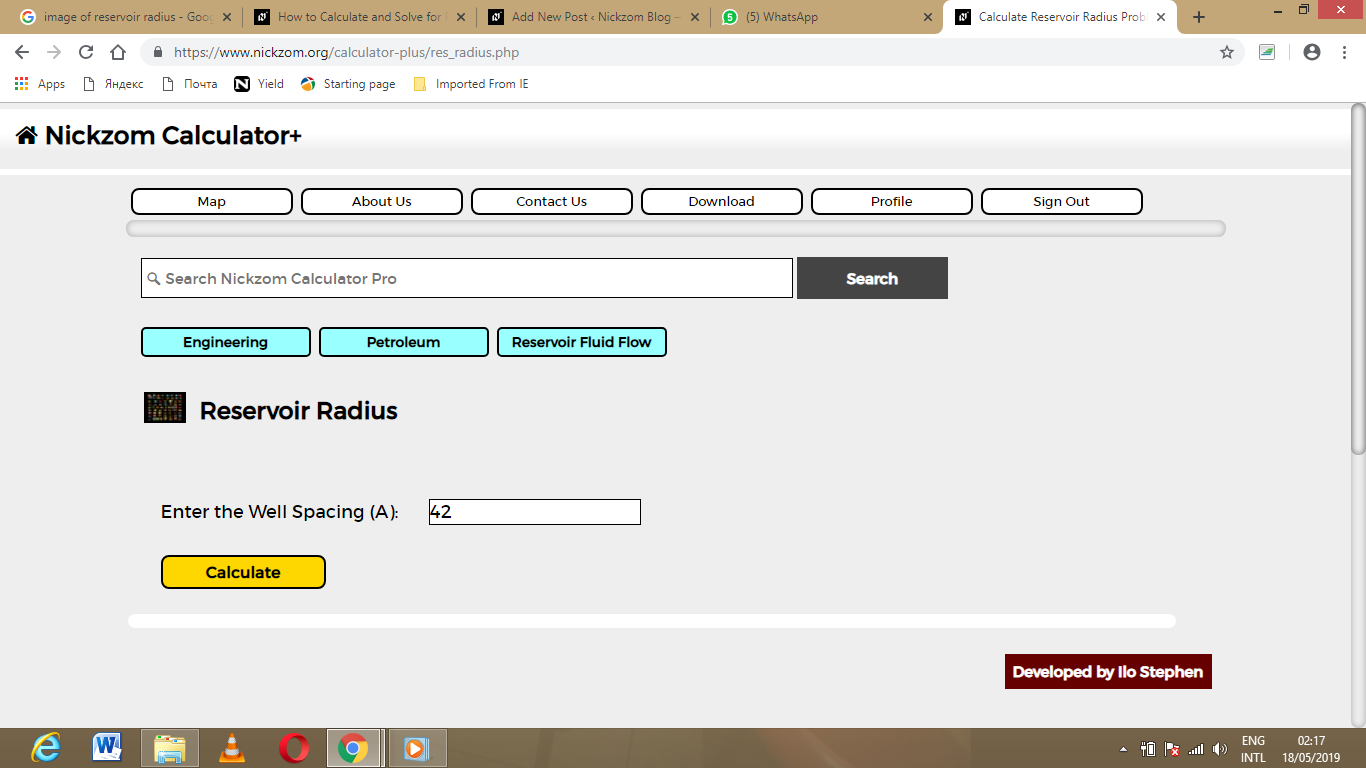Finally, Click on Calculate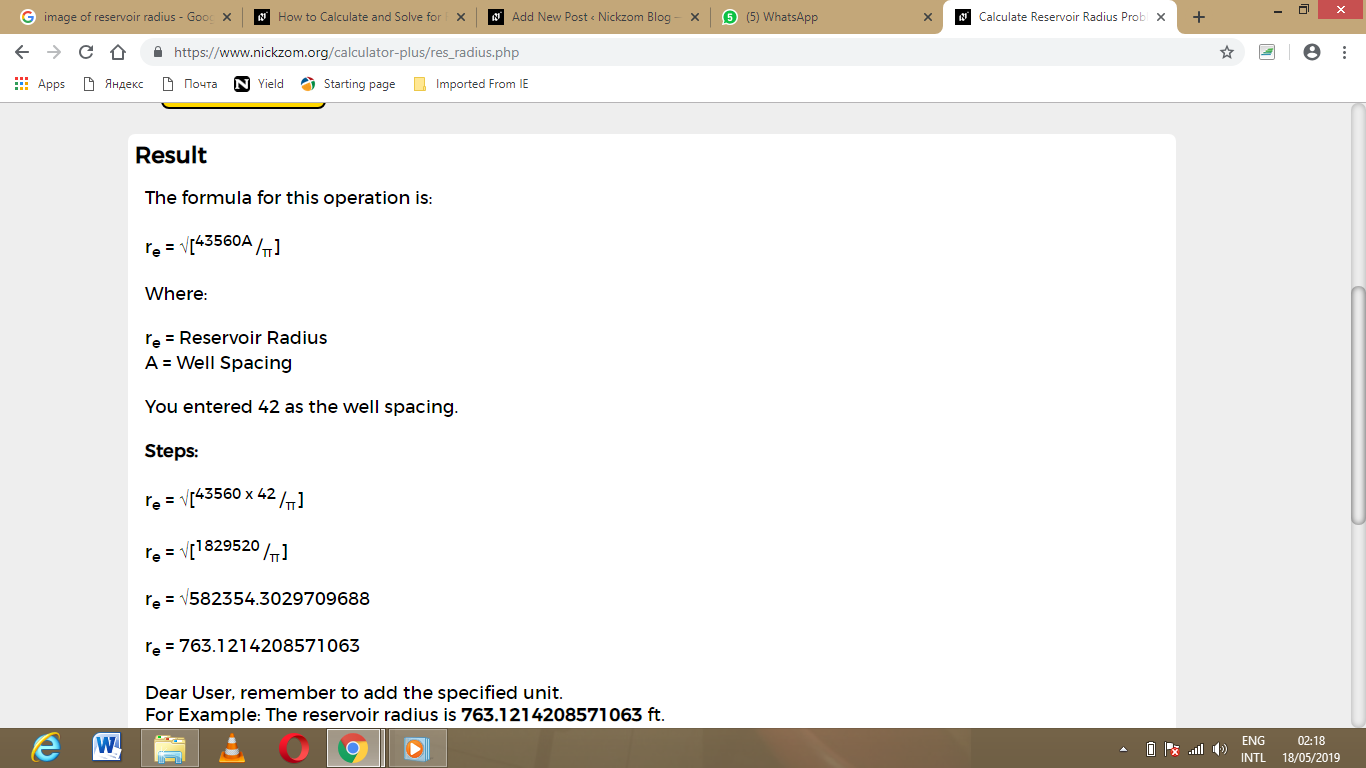As you can see from the screenshot above, Nickzom Calculator – The Calculator Encyclopedia solves for the reservoir radius and presents the formula, workings and steps too.